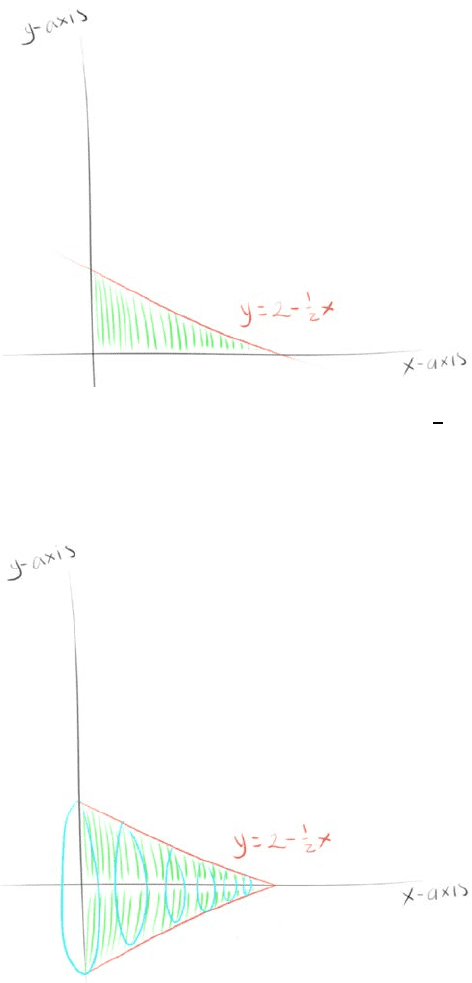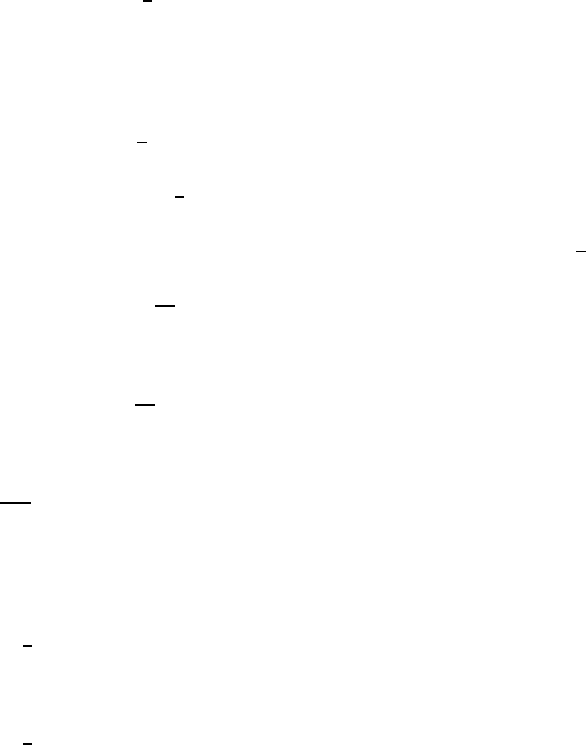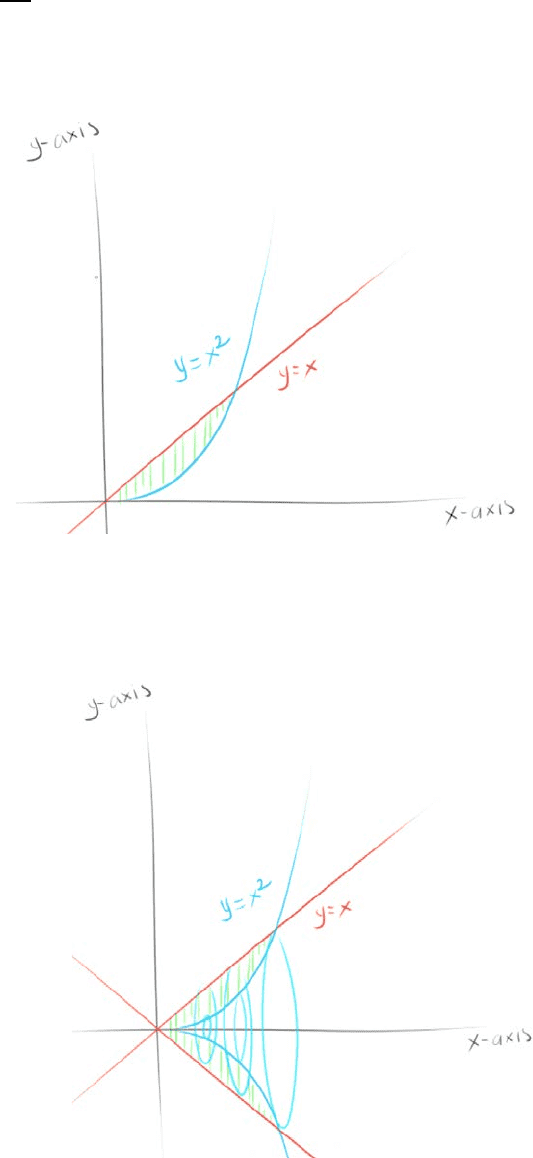Study Guides (390,000)
US (220,000)
UC-Irvine (3,000)
MATH (600)
MATH 2B (100)
All (60)
Midterm

# MATH 2B Midterm: MATH2B, Chapter 6.1 term test 2Premium

Department
Mathematics
Course Code
MATH 2B
Professor
All
Study Guide
Midterm

This preview shows pages 1-3. to view the full 12 pages of the document.Section 6.2 Volumes
Volume
How would you take the 3D volume of a 2D function?
Rotate it around a line
Example
How would you take the volume of the area of = 2 1
2, 
around the x-axis?
What would it look like?
The volume created by the area rotated around the x-axis looks like a cone

Only pages 1-3 are available for preview. Some parts have been intentionally blurred.

Subscribers OnlyHow can we find the volume?
You can use integrals to find the volume of the cone
What is the area of a circle?
2
What would the boundaries be?
The x and y intercepts are where the function intercepts with the other
lines
So = 0, = 4
The function itself is the radius
= (2 1
2)
= 2
= 4
0(2 1
2)2
= 4
04 2 + 1
42
Here, you can move outside the integral and foil out (2 1
2)2
= (4 2 + 1
123) ( 0  4)
Then take the integral
= (16 16 +64
12) (0 0 + 0)
Plugging in the boundaries
= 16
3
Now you can check whether this is true using the actual formula for Volume of a cone
= 1
32
Plug in the necessary components
= 1
3()(2)2(4)

Only pages 1-3 are available for preview. Some parts have been intentionally blurred.

Subscribers Only= 16
3
Example # 1
Find the volume between the curves = and = 2 rotated around the 
What would it look like?